Home » A Guide To Conduct Analysis Using Non-Parametric Statistical Tests

# A Guide To Conduct Analysis Using Non-Parametric Statistical Tests

## Introduction

The average salary package of an economics honors graduate at Hansraj College during the end of the 1980s was around INR 1,000,000 p.a. The number is significantly higher than people graduating in early 80s or early 90s.

What could be the reason for such a high average? Well, one of the highest paid Indian celebrity, Shahrukh Khan graduated from Hansraj College in 1988 where he was pursuing economics honors.

This, and many such examples tell us that average is not a good indicator of the center of the data. It can be extremely influenced by Outliers. In such cases, looking at median is a better choice. It is a better indicator of the center of the data because half of the data lies below the median and the other half lies above it.

So far, so good – I am sure you have seen people make this point earlier. The problem is no one tells you how to perform the analysis like hypothesis testing taking median into consideration.

Statistical tests are used for making decisions. To perform analysis using median, we need to use non-parametric tests. Non-parametric tests are distribution independent tests whereas parametric tests assume that the data is normally distributed. It would not be wrong to say parametric tests are more infamous than non-parametric tests but the former does not take median into account while the latter makes use of median to conduct the analysis.

Without wasting any more time, let’s dive into the world of non-parametric tests.

Note: This article assumes that you have prerequisite knowledge of hypothesis testing, parametric tests, one-tailed & two-tailed tests.

1. How are non-parametric tests different from parametric tests?
2. When can I apply non-parametric tests?
3. Pros and Cons of non-parametric tests
4. Hypothesis testing with non-parametric tests.
5. Non-parametric test
1. Mann Whitney U Test
2. Wilcoxon Sign-Rank Test
3. Sign Test
4. Kruskal-Wallis Test
5. Spearman Rank Correlation

## 1. How are Non-Parametric tests different from Parametric tests?

If you read our articles on probability distributions and hypothesis testing, I am sure you know that there are several assumptions attached to each probability distribution.

Parametric tests are used when the information about the population parameters is completely known whereas non-parametric tests are used when there is no or few information available about the population parameters. In simple words, parametric test assumes that the data is normally distributed. However, non-parametric tests make no assumptions about the distribution of data.

But what are parameters? Parameters are nothing but characteristics of the population that can’t be changed. Let’s look at an example to understand this better.

A teacher calculated average marks scored by the students of her class by using the formula shown below: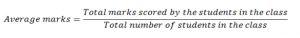Look at the formula given above, the teacher has considered the marks of all the students while calculating total marks. Assuming that the marking of students is done accurately and there are no missing scores, can you change the total marks scored by the students? No. Therefore, average marks is called a parameter of the population since it cannot be changed.

## 2. When can I apply non-parametric tests?

Let’s look at some examples.

1. A winner of the race is decided by the rank and rank is allotted on the basis of crossing the finish line. Now, the first person to cross the finish line is ranked 1, the second person to cross the finish line is ranked 2 and so on. We don’t know by what distance the winner beat the other person so the difference is not known.

2. A sample of 20 people followed a course of treatment and their symptoms were noted by conducting a survey. The patient was asked to choose among the 5 categories after following the course of treatment. The survey looked somewhat like this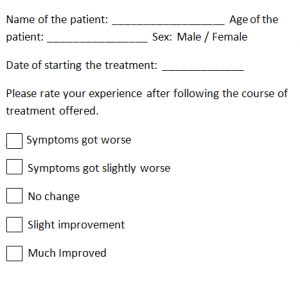Now, if you look carefully the values in the above survey aren’t scalable, it is based on the experience of the patient. Also, the ranks are allocated and not calculated. In such cases, parametric tests become invalid.

For a nominal data, there does not exist any parametric test.

3. Limit of detection is the lowest quantity of a substance that can be detected with a given analytical method but not necessarily quantitated as an exact value. For instance, a viral load is the amount of HIV in your blood. A viral load can either be beyond the limit of detection or it can a higher value.

4. In the example above of average salary package, Shahrukh’s income would be an outlier. What is an outlier? The income of Shahrukh lies at an abnormal distance from the income of other economics graduates. So the income of Shahrukh here becomes an outlier because it lies at an abnormal distance from other values in the data.

To summarize, non-parametric tests can be applied to situations when:

1. The data does not follow any probability distribution
2. The data constitutes of ordinal values or ranks
3. There are outliers in the data
4. The data has a limit of detection

The point to be noted here is that if there exists a parametric test for a problem then using nonparametric tests will yield highly inaccurate answers.

## 3. Pros and Cons of using non-parametric test

In the above discussion, you may have noticed that I mentioned few points where using non-parametric tests could be beneficial or disadvantageous so now let’s look at these points collectively.

### Pros

The pros of using non-parametric tests over parametric tests are

1. Non-parametric tests deliver accurate results even when the sample size is small.

2. Non-parametric tests are more powerful than parametric tests when the assumptions of normality have been violated.

3. They are suitable for all data types, such as nominal, ordinal, interval or the data which has outliers.

### Cons

1. If there exists any parametric test for a data then using non-parametric test could be a terrible blunder.

2. The critical value tables for non-parametric tests are not included in many computer software packages so these tests require more manual calculations.

## 4. Hypothesis testing with non-parametric tests

Now you know that non-parametric tests are indifferent to the population parameters so it does not make any assumptions about the mean, standard deviation etc of the parent population. The null hypothesis here is as general as the two given populations are equal.

Steps to follow while conducting non-parametric tests:

### 1. The first step is to set up hypothesis and opt a level of significance

Now, let’s look at what these two are

Hypothesis: My prediction is that Rahul is going to win the race and the other possible outcome is that Rahul isn’t going to win the race. These are our hypothesis. Our alternative hypothesis is Rahul will win the race because we set alternative hypothesis equal to what we want to prove. The null hypothesis is the opposite one, generally null hypothesis is the statement of no difference. For example,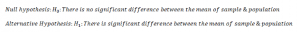Level of significance: It is the probability of making a wrong decision. In the above hypothesis statement, null hypothesis indicates no difference between sample and population mean. Say there’s a 5% risk of rejecting the null hypothesis when there is no difference between the sample and population mean. This risk or probability of rejecting the null hypothesis when it’s true is called level of significance.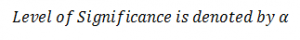In a non-parametric test, the test hypothesis can be one tailed or two tailed depending on the interest of research.

### 2. Set a test statistic

To understand what a statistic is, let’s look at an example. A teacher computed the average marks, say 36, scored by the students in section A, and she used the average marks scored by the students in section A to represent the average marks scored by the students in sections B, C and D. The point to be noted here is that the teacher did not make the use of total marks scored by the students in all the sections instead she used the average marks of section A. Here, the average marks is called a statistic since the teacher did not make use of the entire data.

In a non-parametric test, the observed sample is converted into ranks and then ranks are treated as a test statistic.

### 3. Set decision rule

A decision rule is just a statement that tells when to reject the null hypothesis.

### 4. Calculate test statistic

In non-parametric tests, we use the ranks to compute the test statistic.

### 5. Compare the test statistic to the decision rule

Here, you’ll be accepting or rejecting your null hypothesis on the basis of comparison.

We will dig deeper into this section while discussing types of non-parametric tests.

## 5. Non-Parametric Tests

### Mann Whitney U test

Also known as Mann Whitney Wilcoxon and Wilcoxon rank sum test and, is an alternative to independent sample t-test. Let’s understand this with the help of an example.

A pharmaceutical organization created a new drug to cure sleepwalking and observed the result on a group of 5 patients after a month. Another group of 5 has been taking the old drug for a month. The organization then asked the individuals to record the number of sleepwalking cases in the last month. The result was: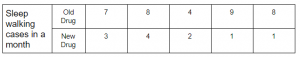If you look at the table, the number of sleepwalking cases recorded in a month while taking the new drug is lower as compared to the cases reported while taking the old drug.

Look at the graphs given below.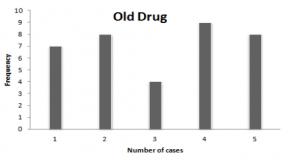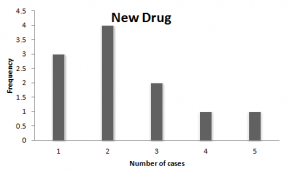Now, here you see that the frequency of sleepwalking cases is lower when the person is taking new drugs.

Understood the problem? Let’s see how Mann Whitney U test works here. We are interested in knowing whether the two groups taking different drugs report the same number of sleepwalking cases or not. The hypothesis is given below: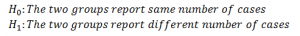I am selecting 5% level of significance for this test. The next step is to set a test statistic.

For Mann Whitney U test, the test statistic is denoted by U which is the minimum of U1 and U2.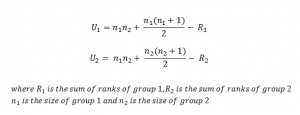Now, we will compute the ranks by combining the two groups. The question is

How to assign ranks?

Ranks are a very important component of non-parametric tests and therefore learning how to assign ranks to a sample is considerably important. Let’s learn how to assign ranks.

1. We will combine the two samples and arrange them in ascending order. I am using OD and ND for Old Drug and New Drug respectively.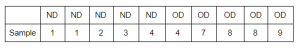The lowest value here is assigned the rank 1 and the second lowest value is assigned the rank 2 and so on.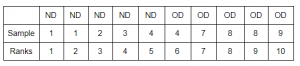But notice that the numbers 1, 4 and 8 are appearing more than once in the combined sample. So the ranks assigned are wrong.

How to assign ranks when there are ties in the sample?

Ties are basically a number appearing more than once in a sample. Look at the position of number 1 in the sample after sorting the data. Here, the number 1 is appearing at 1st and 2nd position. In such a case, we take the mean of 1 and 2 (because the number 1 is appearing at 1st and 2nd position) and assign the mean to the number 1 as shown below. We follow the same steps for number 4 and 8. The number 4 here is appearing at position 5th and 6th and their mean is 5.5 so we assign rank 5.5 to the number 4. Calculate rank for number 8 along these lines.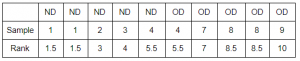We assign the mean rank when there are ties in a sample to make sure that the sum of ranks in each sample of size n is same. Therefore, the sum of ranks will always be equal to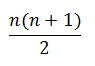2. The next step is to compute the sum of ranks for group 1 and group 2.

R1 = 15.5
R2 = 39.5

3. Using the formula of U1 & U2, compute their values.

U1 = 24.5
U2 = 0.5

Now, U = min(U1, U2) = 0.5

Note: For Mann Whitney U test, the value of U lies in the range(0, n1*n2) where 0 indicates that the two groups are completely different from each other and n1*n2 indicates some relation between the two groups. Also, U1 + U2 is always equal to n1*n2. Notice that the value of U is 0.5 here which is very close to 0.

Now, we determine a critical value (denoted by p), using the table for critical values, which is a point derived from the level of significance of the test and is used to reject or accept the null hypothesis. In Mann Whitney U test, the test criteria are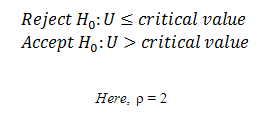U < critical value, therefore, we reject the null hypothesis and conclude that the there’s no significant evidence to state that two groups report same number of sleepwalking cases.

### Wilcoxon Sign-Rank Test

This test can be used in place of paired t-test whenever the sample violates the assumptions of a normal distribution.

A teacher taught a new topic in the class and decided to take a surprise test on the next day. The marks out of 10 scored by 6 students were as follows:

Note: Assume that the following data violates the assumptions of normal distribution.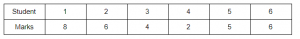Now, the teacher decided to take the test again after a week of self-practice. The scores were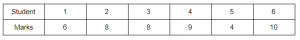Let’s check if the marks of the students have improved after a week of self-practice.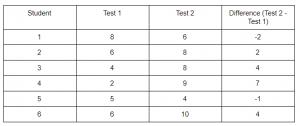In the table above, there are some cases where the students scored less than they scored before and in some cases, the improvement is relatively high (Student 4). This could be due to random effects. We will analyse if the difference is systematic or due to chance using this test.

The next step is to assign ranks to the absolute value of differences. Note that this can only be done after arranging the data in ascending order.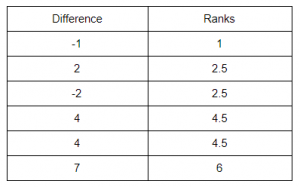In Wilcoxon sign-rank test, we need signed ranks which basically is assigning the sign associated with the difference to the rank as shown below.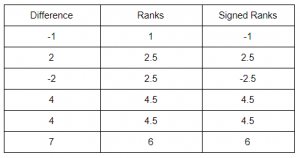Easy, right? Now, what is the hypothesis here?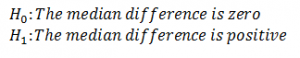The hypothesis can be one-sided or two-sided and I am considering one-sided hypothesis and using 5% level of significance. Therefore,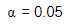The test statistic for this test is W is the smaller of W1 and W2 defined below: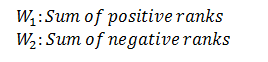W1 = 17.5
W2 = 3.5
W = min(W1, W2 ) = 3.5

Here, if W1 is similar to W2 then we accept the null hypothesis. Otherwise, in this example, if the difference reflects greater improvement in the marks scored by the students, then we reject the null hypothesis.

The critical value of W can be looked up in the table.

The criteria to accept or reject null hypothesis are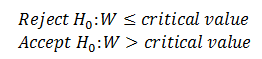Here, W > critical value=2, therefore we accept the null hypothesis and conclude that there’s no significant difference between the marks of two tests.

### Sign Test

This test is similar to Wilcoxon sign-rank test and this can also be used in place of paired t-test if the data violates the assumptions of normality. I am going to use the same example that I used in Wilcoxon sign-rank test, assuming that it does not follow the normal distribution, to explain sign test.

Let’s look at the data again.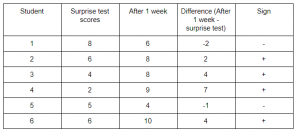In sign test, we don’t take magnitude into consideration thereby ignoring the ranks. The hypothesis is same as before.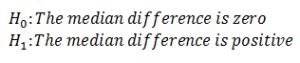Here, if we see a similar number of positive and negative differences then the null hypothesis is true. Otherwise, if we see more of positive signs then the null hypothesis is false.

Test Statistic:  The test statistic here is smaller of the number of positive and negative signs.

Determine the critical value and the criteria for rejecting or accepting null hypothesis is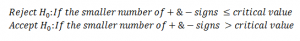Here, the smaller number of + & – signs = 2 < critical value = 6. Therefore, we reject the null hypothesis and conclude that there’s no significant evidence to state that the median difference is zero.

### Kruskal-Wallis Test

This test is extremely useful when you are dealing with more than 2 independent groups and it compares median among k populations. This test is an alternative to One way ANOVA when the data violates the assumptions of normal distribution and when the sample size is too small.

Note: The Kruskal-Wallis test can be used for both continuous and ordinal-level dependent variables.

Let’s look at an example to enhance our understanding of Kruskal-Wallis test.

Patients suffering from Dengue were divided into 3 groups and three different types of treatment were given to them. The platelet count of the patients after following a 3-day course of treatment is given below.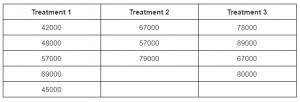Notice that the sample size is different for the three treatments which can be tackled using Kruskal-Wallis test.

Sample sizes for treatments 1, 2 and 3 are as follows:

Treatment 1; n1 = 5
Treatment 2; n2 = 3
Treatment 3; n3 = 4
n = n1 + n2 + n3 = 5+3+4 = 12

The hypothesis here is given below and I have selected 5% level of significance.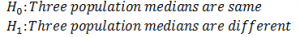Order these samples from smallest to largest and then assign ranks to the clubbed sample.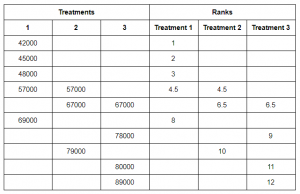Recall that the sum of ranks will always be equal to n(n+1)/2.

Here, sum of ranks = 78
n(n+1)/2 = (12*13)/2 = 78

We have to check if there is a difference between 3 population medians so we will summarize the sample information in a test statistic based on ranks. Here, the test statistic is denoted by H and given by the following formula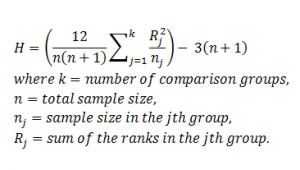The next step is to determine the critical value of H using the table of critical values and the test criteria is given by: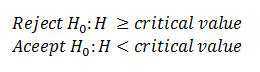H comes out to be 6.0778 and the critical value is 5.656. Therefore, we reject our null hypothesis and conclude that there’s no significant evidence to state that the three population medians are same.

Note: In a Kruskal-Wallis test, if there are 3 or more independent comparison groups with 5 or more observations in each group then the test statistic H approximates a chi-square distribution with k-1 degree of freedom. Therefore, in such a case, you can find the critical value of the test in the chi-square distribution table for critical values.

### Spearman Rank Correlation

I went to the market to buy a skirt and coincidently my friend bought the same skirt from the market near her place but she paid a higher price for it. The market near my friend’s place is more expensive as compared to mine. So does a region affect the price of a commodity? If it does then there is a link between the region and price of the commodity. We make use of Spearman rank correlation here because it establish if there is the correlation between two datasets.

The prices of vegetables differ across areas. We can check if there’s a relation between the price of a vegetable and area by using Spearman rank correlation. The hypothesis here is: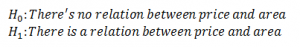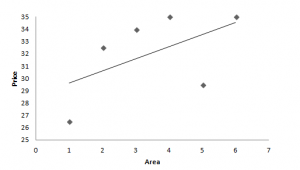Here, the trend line suggests a positive correlation between the price of vegetable and area. However, Spearman’s rank correlation method should be used to check the direction and strength of correlation.

Spearman rank correlation is a non-parametric alternative to Pearson correlation coefficient and is denoted by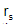. The value oflies in the range (-1,1) where
-1 represents a negative correlation between ranks
0 represents no correlation between ranks
1 represents a positive correlation between ranks

After assigning ranks to the sample, use the following formula to calculate Spearman rank correlation coefficient.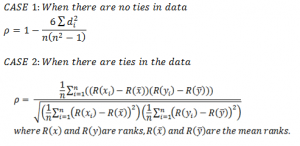Let’s understand the application of these formula using a sample data.The following table includes marks of students in math and science.
The null hypothesis states that there is no relationship between the marks and alternative hypothesis states that there is a relationship between the marks. I have selected 5% level of significance for this test.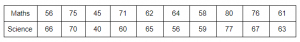Now calculate rank and d which is the difference between ranks and n is the sample size = 10. This is done as follows: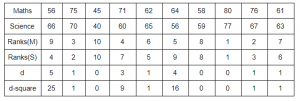Now, use the formula to calculate Spearman rank correlation coefficient. Hence, the Spearman rank correlation comes out to be 0.67 which indicates a positive relation between the ranks students obtained in maths and science test which implies that the higher you ranked in maths, the higher you ranked in science and vice-versa.

You can also check this by determining the critical values using the significance level and sample size. The criteria to reject or accept null hypothesis is given by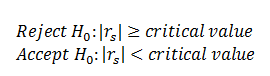Note: The degree of freedom here is n-2.
The critical value is found to be 0.033 which is lower than 0.67. Hence, we reject our null hypothesis.

## End Notes

Non-parametric tests are more powerful when the assumptions for parametric tests are violated and can be used for all data types such as nominal, ordinal, interval and also when data has outliers. If any of the parametric tests is valid for a problem then using non-parametric test will give highly inaccurate results.

To summarize,

Mann Whitney U test is used for testing the difference between two independent groups with ordinal or continuous dependent variable.

Wilcoxon sign rank test is used for testing the difference between two related variables which takes into account the magnitude and direction of difference, however, Sign test ignores the magnitude and only considers the direction of the difference.

Kruskal-Wallis test compares the outcome among more than 2 independent groups by making use of the medians.

Spearman Rank Correlation technique is used to check if there is a relationship between the two data sets and it also tells about the type of relationship.

I hope that you find this article useful and if you would like to see more articles on non-parametric or parametric tests then write down in the comment section below.

### Learn, engage,compete, and get hired!

You can also read this article on our Mobile APP•Arun Vaibhav says:

Incredibly Awesome Article Ms. Nijhawan!!!!
Very lucid, clear, crisp and nicely organized.
This cleared my understanding on Non-Parametric tests.
I would recommend, if you can. please post, R or SAS codes for the same,

Thanks a lot!! Really appreciate the effort!

Thanks.

•Vaibhav123 says:

That being said, primarily in order to decide whether to use parametric or non-parametric test, we need to test Normality of the data.
If a data has several variables (assume 20) and if we want to test the independence, first, how can we test the Normality and second, what test from aforesaid tests is preferred?

Thanks,
Vaibhav

•Raghav Agrawal says:

•pradeep kr says:

Super

•Himani says:

Great job. Such a nice artile radhika 🙂

•Margie says:

Well done!

•Yunus says:

Great Post!!

Why not use bootstrapping and infer difference from bootstrap sample distribution?
this does not require any assumption of population distribution and hence non parametric.
I usually do these steps:

1) Combine the results from the different groups in a single data set.
2) Randomly draw (with replacement) a resample of the same size as group A.
3) Randomly draw (with replacement) a resample of the same size as group B.
4) Whatever statistic or estimate was calculated for the original samples (e.g., difference in group proportions), calculate it now for the resamples, and record; this constitutes one iteration.
5) Repeat the previous steps R times to yield a distribution of the test statistic.

Infer about the differences from the distribution above. I can post the R(or python ) code for the above if required, but that is fairly straighforward.